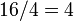# Center of nontrivial semidirect product of Z4 and Z4

This article is about a particular subgroup in a group, up to equivalence of subgroups (i.e., an isomorphism of groups that induces the corresponding isomorphism of subgroups). The subgroup is (up to isomorphism) Klein four-group and the group is (up to isomorphism) nontrivial semidirect product of Z4 and Z4 (see subgroup structure of nontrivial semidirect product of Z4 and Z4).
The subgroup is a normal subgroup and the quotient group is isomorphic to Klein four-group.
VIEW: Group-subgroup pairs with the same subgroup part | Group-subgroup pairs with the same group part| Group-subgroup pairs with the same quotient part | All pages on particular subgroups in groups

## Definition

Consider the group:$G := \langle x,y \mid x^4 = y^4 = e, yxy^{-1} = x^3 \rangle$.

This is a group of order 16 with elements:$\! \{ e,x,x^2,x^3,y,xy,x^2y,x^3y,y^2,xy^2,x^2y^2,x^3y^2,y^3,xy^3,x^2y^3,x^3y^3 \}$

We are interested in the subgroup:$\! H = \{ e, x^2, y^2, x^2y^2 \}$

This is the center. In particular, it is a normal subgroup isomorphic to the Klein four-group and the quotient group is also isomorphic to the Klein four-group.

The multiplication table for$H$ is given as follows:

Element/element$e$$x^2$$y^2$$x^2y^2$$e$$e$$x^2$$y^2$$x^2y^2$$x^2$$x^2$$e$$x^2y^2$$y^2$$y^2$$y^2$$x^2y^2$$e$$x^2$$x^2y^2$$x^2y^2$$y^2$$x^2$$e$

## Cosets$H$ is a normal subgroup, so its left cosets coincide with its right cosets. There are four cosets, because the index of$H$ in$G$ is$16/4 = 4$. These are:$\{ e, x^2, y^2, x^2y^2 \}, \{ x, x^3, xy^2, x^3y^2 \}, \{ y, x^2y, y^3, x^2y^3 \}, \{ xy, x^3y, xy^3, x^3y^3 \}$

The quotient group is isomorphic to a Klein four-group, and the multiplication table is given as follows:

## Subgroup-defining functions

Subgroup-defining function Meaning in general Why it takes this value GAP verification (set G := SmallGroup(16,4); H := Center(G); before starting)
center elements that commute with every group element Follows from multiplication table Definitional
socle join of all minimal normal subgroups, equivalently$\Omega_1(Z(G))$ The minimal normal subgroups are$\{ e, x^2 \}$ (see here),$\{ e, y^2 \}$ (see here),$\{ e, x^2y^2 \}$ (see here). H = Socle(G); using Socle
first omega subgroup generated by the set of all elements of order$p$ (here$p = 2$) The elements of order 2 are precisely$x^2, y^2, x^2y^2$.
[[arises as subgroup-defining function::agemo subgroups of group of prime power order|first agemo subgroup generated by the set of all$p^{th}$ powers (here$p = 2$, so squares) The squares are precisely$e, x^2, y^2$ (note$x^2y^2$ is not a square, but is in the subgroup generated). H = Agemo(G,2,1); using Agemo

## Subgroup properties

### Invariance under automorphisms and endomorphisms

Property Meaning Satisfied? Explanation GAP verification (set G := SmallGroup(16,4); H := Center(G); first) -- see #GAP implementation
normal subgroup invariant under inner automorphisms Yes center is normal IsNormal(G,H); using IsNormal
characteristic subgroup invariant under all automorphisms Yes center is characteristic IsCharacteristicSubgroup(G,H); using IsCharacteristicSubgroup
fully invariant subgroup invariant under all endomorphisms Yes omega subgroups are fully invariant, agemo subgroups are fully invariant IsFullinvariant(G,H); using IsFullinvariant
verbal subgroup generated by set of words Yes agemo subgroups are verbal
isomorph-free subgroup no other isomorphic subgroup Yes precisely the set of elements of order two (plus the identity element).
homomorph-containing subgroup contains any homomorphic image Yes precisely the set of elements of order two (plus the identity element).

## GAP implementation

The group and subgroup pair can be constructed using GAP's SmallGroup and Center functions:

G := SmallGroup(16,4); H := Center(G);

Here is the GAP display:

gap> G := SmallGroup(16,4); H := Center(G);
<pc group of size 16 with 4 generators>
Group([ f3, f4 ])

Here is some GAP code to verify the assertions on this page:

gap> Order(G);
16
gap> Order(H);
4
gap> Index(G,H);
4
gap> StructureDescription(H);
"C2 x C2"
gap> StructureDescription(G/H);
"C2 x C2"
gap> H = Socle(G);
true
gap> H = Agemo(G,2,1);
true
gap> IsNormal(G,H);
true
gap> IsCharacteristicSubgroup(G,H);
true
gap> IsFullinvariant(G,H);
true